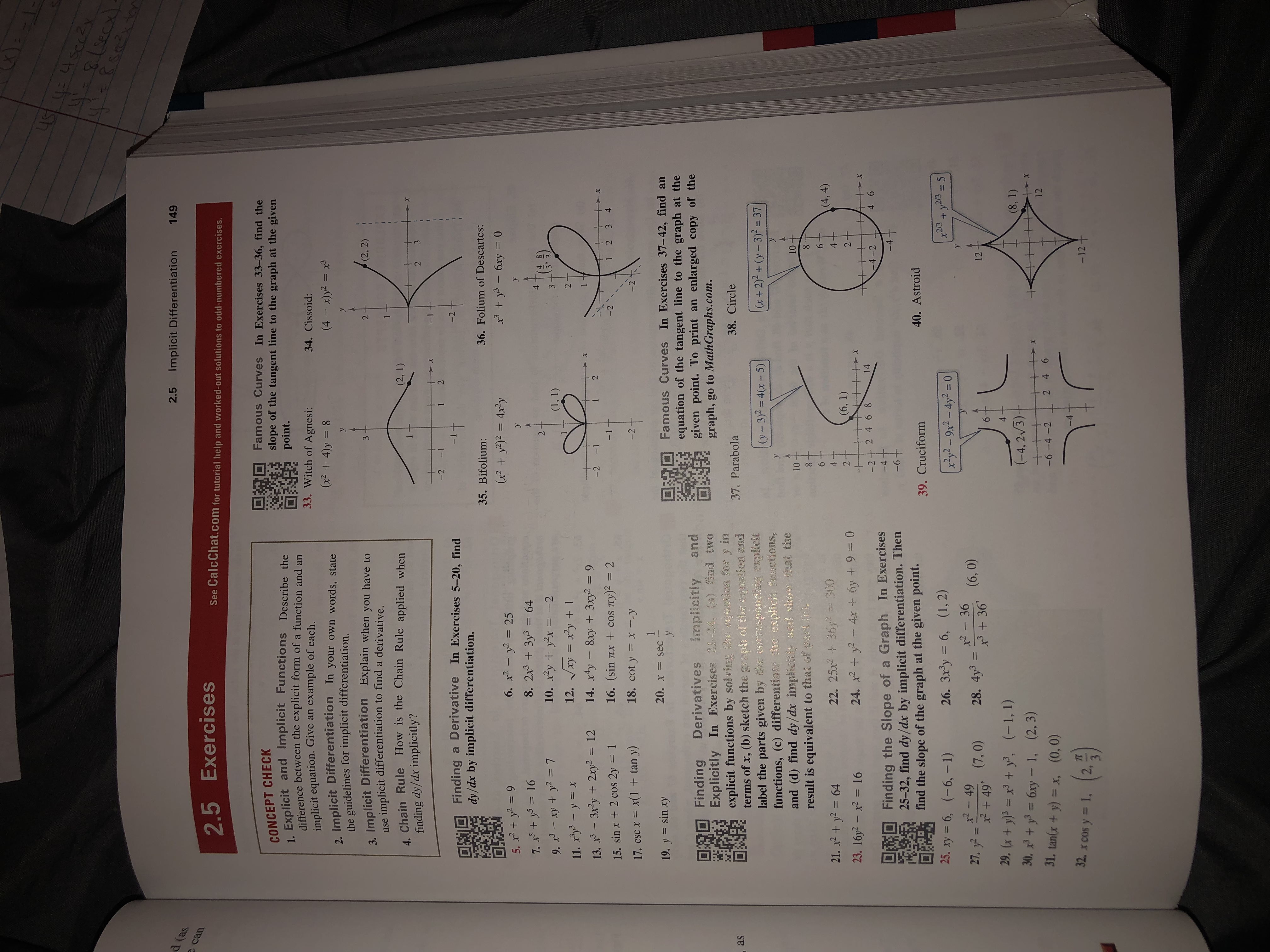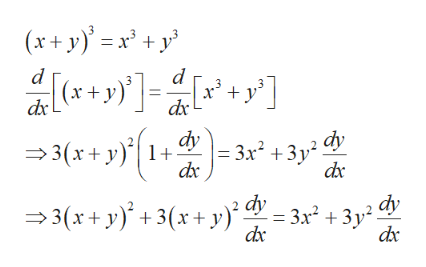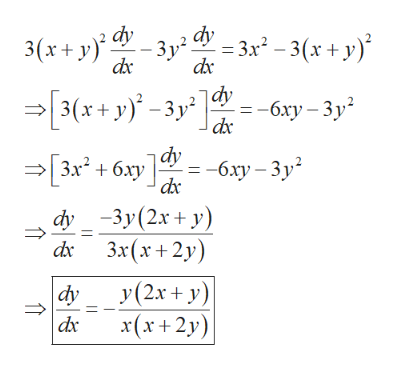# uSsecఇపె2.5Implicit Differentiation149d (ascan2.5 ExercisesSee CalcChat.com for tutorial help and worked-out solutions to odd-numbered exercises.Famous Curves In Exercises 33-36, find theslope of the tangent line to the graph at the givenpoint.CONCEPT CHECK1. Explicit and Implicit Functions Describe thedifference between the explicit form of a function and an33. Witch of Agnesi:34. Cissoid:implicit equation. Give an example of each.(x2 4)y = 8(4 x)y2 = x32. Implicit Differentiationthe guidelines for implicit differentiation.Implicit Differentiation Explain when you have toimplicit differentiation to find a derivative.1In your own words, stateyy(2, 2)23useChain Rule How is the Chain Rule applied when(2, 1)finding dy/dx implicitly?322-2 -1Derivative In Exercises 5-20, find-2Findingdy/dx by implicit differentiation.36. Folium of Descartes:35. Bifolium:3y36xy 0(x2 y2) = 4x2y6. x2-y2255. x+297. xy5 169. x3-y2118. 2x3+3y3= 64410. xyy2x -212. Vxy = xy + 173(1, 1)211. x'y-y x14. xty 8xy+3xy2 =9ry) 213. x-32y2xy2 122-1-2X16. (sin4321TX +cOS15. sinx +2 cos 2y18. cot y-2x --y17. csC x x(1 + tan y)120. x sec -yFamous Curves In Exercises 37-42, find anequation of the tangent line to the graph at thegiven point. To print angraph, go to Math Graphs.com.19. y = sinxyandenlarged copy of theFinding DerivativesExplicitly In Exercises 31-24, (a) find twoexplicit functions by solving he cquntion fer y interms of x, (b) sketch the grapt of the cquatken andlabel the parts given by orraspondisg srxplicitfunctions, (c) differentiadhe explicit fnctions,and (d) find dy/dx implicisy and show ihat theresult is equivalent to that of park (c).Implicitly38. Circle37. Parabolaas(x+ 2)2+(y-3)2= 37(y-3)2= 4(x-5)1010(4, 4)22. 25x236y 30021. x2+ y2 6423. 16y2- 2= 16(6, 1)4x + 6y +9= 024. x2y2+14-2+ 2 46 84 6-4-2Finding the Slope of a Graph In Exercises25-32, find dy/dx by implicit differentiation. Thenfind the slope of the graph at the given point.-4-640. Astroid39. Cruciformx2/3y2/3525. xy = 6, (-6, - 1)26. 3x3y = 6, (1, 2)x2y2- 9x2-4y2 - 0x2-36x2-4927. y2x 49' (7,0)29. (x + y)3 = x3 + y, (-1, 1)30. y6xy - 1, (2, 3)28. 4y3111236 (6,0)4(8, 1)(-4, 2/3)+2 46Xx1231. tan(x+y) = x,-6-4 -2(0, 0)-4(a9)32. x COS y = 1,-12+2,34 21

Question
3 views

29help_outlineImage TranscriptioncloseuS sec ఇపె 2.5 Implicit Differentiation 149 d (as can 2.5 Exercises See CalcChat.com for tutorial help and worked-out solutions to odd-numbered exercises. Famous Curves In Exercises 33-36, find the slope of the tangent line to the graph at the given point. CONCEPT CHECK 1. Explicit and Implicit Functions Describe the difference between the explicit form of a function and an 33. Witch of Agnesi: 34. Cissoid: implicit equation. Give an example of each. (x2 4)y = 8 (4 x)y2 = x3 2. Implicit Differentiation the guidelines for implicit differentiation. Implicit Differentiation Explain when you have to implicit differentiation to find a derivative. 1 In your own words, state y y (2, 2) 2 3 use Chain Rule How is the Chain Rule applied when (2, 1) finding dy/dx implicitly? 3 2 2 -2 -1 Derivative In Exercises 5-20, find -2 Finding dy/dx by implicit differentiation. 36. Folium of Descartes: 35. Bifolium: 3y36xy 0 (x2 y2) = 4x2y 6. x2-y225 5. x+29 7. xy5 16 9. x3-y2 11 8. 2x3+3y3= 64 4 10. xyy2x -2 12. Vxy = xy + 1 7 3 (1, 1) 2 11. x'y-y x 14. xty 8xy+3xy2 =9 ry) 2 13. x-32y2xy2 12 2 -1 -2 X 16. (sin 4 3 2 1 TX +cOS 15. sinx +2 cos 2y 18. cot y -2 x --y 17. csC x x(1 + tan y) 1 20. x sec - y Famous Curves In Exercises 37-42, find an equation of the tangent line to the graph at the given point. To print an graph, go to Math Graphs.com. 19. y = sinxy and enlarged copy of the Finding Derivatives Explicitly In Exercises 31-24, (a) find two explicit functions by solving he cquntion fer y in terms of x, (b) sketch the grapt of the cquatken and label the parts given by orraspondisg srxplicit functions, (c) differentiadhe explicit fnctions, and (d) find dy/dx implicisy and show ihat the result is equivalent to that of park (c). Implicitly 38. Circle 37. Parabola as (x+ 2)2+(y-3)2= 37 (y-3)2= 4(x-5) 10 10 (4, 4) 22. 25x236y 300 21. x2+ y2 64 23. 16y2- 2= 16 (6, 1) 4x + 6y +9= 0 24. x2y2 + 14 -2+ 2 46 8 4 6 -4-2 Finding the Slope of a Graph In Exercises 25-32, find dy/dx by implicit differentiation. Then find the slope of the graph at the given point. -4 -6 40. Astroid 39. Cruciform x2/3y2/35 25. xy = 6, (-6, - 1) 26. 3x3y = 6, (1, 2) x2y2- 9x2-4y2 - 0 x2-36 x2-49 27. y2 x 49' (7,0) 29. (x + y)3 = x3 + y, (-1, 1) 30. y6xy - 1, (2, 3) 28. 4y3 11 12 36 (6,0) 4 (8, 1) (-4, 2/3) + 2 46 Xx 12 31. tan(x+y) = x, -6-4 -2 (0, 0) -4 (a9) 32. x COS y = 1, -12+ 2, 3 4 2 1 fullscreen
check_circle

Step 1

Obtain dy/dx by implicit differentiation as follows.help_outlineImage Transcriptionclose(x+y)x+y d d (x+y) dx dx dy 3(x+y1+ 3x3y2 dx dy 3(xy)3(r+y)3x2 +3y dc fullscreen
Step 2

Simplify fur...help_outlineImage Transcriptionclosedy -Зу -3г - 3(х+ у) 3(x+ у). dx - 3(х+у) -Зу - бху — Зу dac 3 3x* + бху gy — бху — Зу dx dy -3у (2х + у) dx Зx(х+2у) У(2х + у) dy x(х+2у) fullscreen

### Want to see the full answer?

See Solution

#### Want to see this answer and more?

Solutions are written by subject experts who are available 24/7. Questions are typically answered within 1 hour.*

See Solution
*Response times may vary by subject and question.
Tagged in

### Derivative